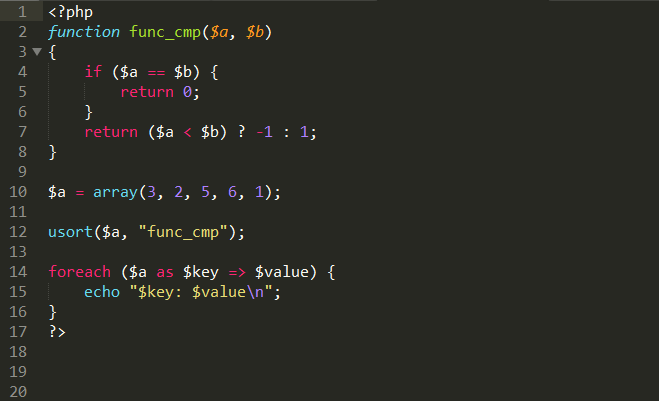functions

# What is usort() function in php & how to use it ?The usort() function sort an array by values using a user-defined comparison function. It accepts two parameters, the first parameter must be an array to sort and the second parameter must be a string that defines a function. The function must return an integer less than, equal to, or greater than zero if the first argument is considered to be respectively less than, equal to, or greater than the second. Note that before PHP 7.0.0 this integer had to be in the range from -2147483648 to 2147483647.

Points to be Noted :
-> If two values compare as equal, their relative order in the sorted array is undefined.
-> This function assigns new keys to the elements in an array. It will remove any existing keys that may have been assigned, rather than just reordering the keys.

Example :

```<?php
function func_cmp(\$a, \$b)
{
if (\$a == \$b) {
return 0;
}
return (\$a < \$b) ? -1 : 1;
}

\$a = array(3, 2, 5, 6, 1);

usort(\$a, "func_cmp");

foreach (\$a as \$key => \$value) {
echo "\$key: \$value\n";
}
?>```

Output :